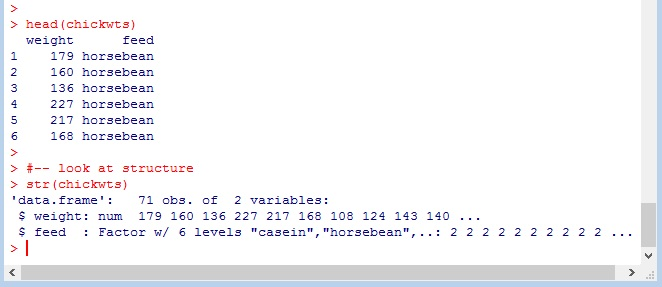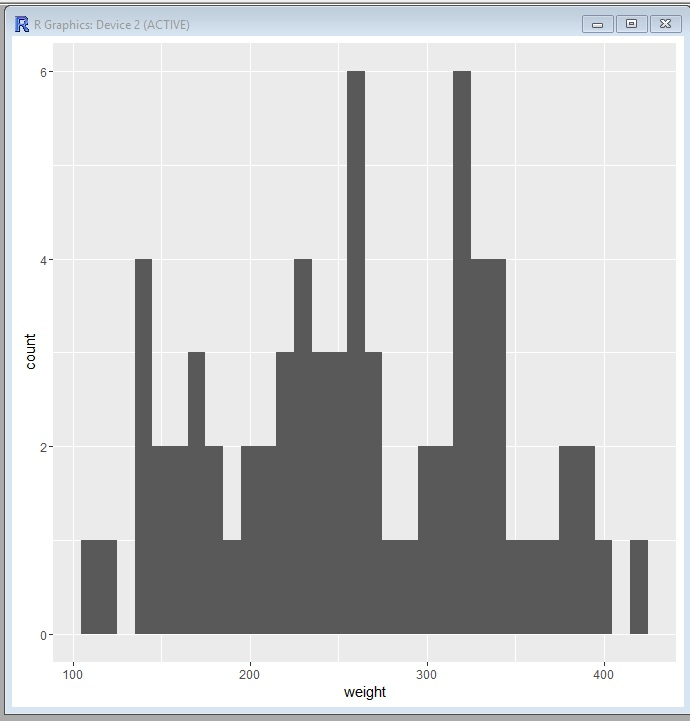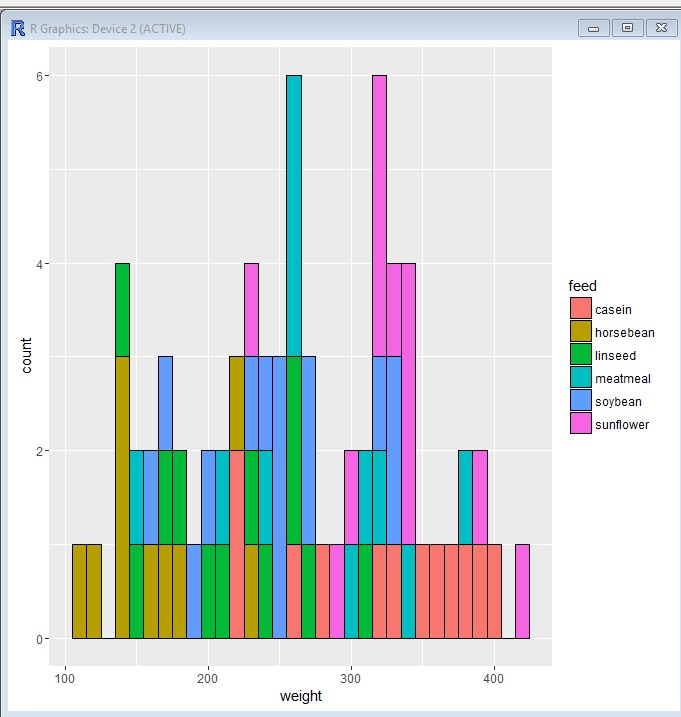# R: ggplot – Histograms

Let’s make a Histogram using ggplot

First step, import the ggplot2 library

`library(ggplot2)`

Now let’s look at our data. In this example I am using chickwts from R Data

```head(chickwts)

str(chickwts)```As you can see above, this data contains 2 columns weight ( numeric) and feed(Factor with 6 levels)

## Histogram

In a histogram, we don’t need to worry about assigning a Y axis, the Y axis is the frequency count of our X variable.

Let’s set our data and x axis in ggplot. Let’s also assign it to variable.

`pl <- ggplot(data=chickwts, aes(x=weight))`

Now we can use the assigned variable in conjunction with our geom()

`pl + geom_histogram(binwidth=10)`

In our geom, I set a binwidth. This tells us how wide to make our bars. Setting binwidth to 10 makes each bar 10 units wide.Let’s give it some color

`pl + geom_histogram(binwidth=10, fill="blue")`Now, let’s set our colors based on our 2nd column “feed”. Note how a legend is automatically generated.

`pl + geom_histogram(binwidth=10, aes(fill=feed))`For the finishing touch, let’s add some black bordering around our boxes.

`pl + geom_histogram(binwidth=10, aes(fill=feed), color="black")`## The Code

```library(ggplot2)

#-- look at structure
str(chickwts)

#set data and x axis value
pl <- ggplot(data=chickwts, aes(x=weight))

#create histogram, binwidth 10
pl + geom_histogram(binwidth=10)

#change bars to blue
pl + geom_histogram(binwidth=10, fill="blue")

#set color of bars by feed column values
pl + geom_histogram(binwidth=10, aes(fill=feed))